# Spheroid

For spheroids in cell culturing, see 3D cell culture.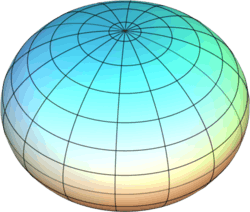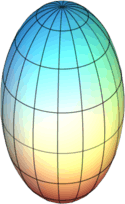oblate prolate

A spheroid, or ellipsoid of revolution, is a quadric surface obtained by rotating an ellipse about one of its principal axes; in other words, an ellipsoid with two equal semi-diameters.

If the ellipse is rotated about its major axis, the result is a prolate (elongated) spheroid, like an American football or rugby ball. If the ellipse is rotated about its minor axis, the result is an oblate (flattened) spheroid, like a lentil. If the generating ellipse is a circle, the result is a sphere. A spheroid has circular symmetry.

Because of the combined effects of gravity and rotation, the Earth's shape is not quite a sphere but instead is slightly flattened in the direction of its axis of rotation. For that reason, in cartography the Earth is often approximated by an oblate spheroid instead of a sphere. The current World Geodetic System model uses a spheroid whose radius is 6,378.137 km (3,963.191 mi) at the equator and 6,356.752 km (3,949.903 mi) at the poles.

The word spheroid originally meant an approximately spherical body, admitting irregularities even beyond the bi- or tri-axial ellipsoidal shape, and that is how it is used in some older papers on geodesy (for example, referring to truncated spherical harmonic expansions of the Earth).

## Equation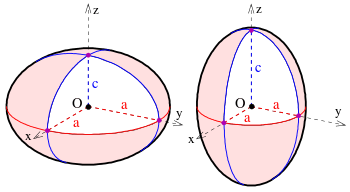The assignment of semi-axes on a spheroid. It is oblate if c < a (left) and prolate if c > a (right).

The equation of a tri-axial ellipsoid centred at the origin with semi-axes a, b and c aligned along the coordinate axes isThe equation of a spheroid with z as the symmetry axis is given by setting a = b:The semi-axis a is the equatorial radius of the spheroid, and c is the distance from centre to pole along the symmetry axis. There are two possible cases:

• c < a: oblate spheroid
• c > a: prolate spheroid

The case of a = c reduces to a sphere.

## Properties

### Area

An oblate spheroid with c < a has surface areaThe oblate spheroid is generated by rotation about the z-axis of an ellipse with semi-major axis a and semi-minor axis c, therefore e may be identified as the eccentricity. (See ellipse.)

A prolate spheroid with c > a has surface areaThe prolate spheroid is generated by rotation about the z-axis of an ellipse with semi-major axis c and semi-minor axis a, therefore e may again be identified as the eccentricity. (See ellipse.) 

These formulas are identical in the sense that the formula for Soblate can be used to calculate the surface area of a prolate spheroid and vice versa. However, e then becomes imaginary and can no longer directly be identified with the eccentricity. Both of these results may be cast into many other forms using standard mathematical identities and relations between parameters of the ellipse.

### Volume

The volume inside a spheroid (of any kind) is /3a2c ≈ 4.19a2c. If A = 2a is the equatorial diameter, and C = 2c is the polar diameter, the volume is π/6A2C ≈ 0.523A2C.

### Curvature

If a spheroid is parameterized aswhere β is the reduced or parametric latitude, λ is the longitude, and π/2 < β < +π/2 and −π < λ < +π, then its Gaussian curvature isand its mean curvature isBoth of these curvatures are always positive, so that every point on a spheroid is elliptic.

### Aspect ratio

The aspect ratio of an oblate spheroid/ellipse, b : a, is the ratio of the polar to equatorial lengths, while the flattening (also called oblateness) f, is the ratio of the equatorial-polar length difference to the equatorial length:These are just two of several different parameters used to define an ellipse and its solid body counterparts.

## Applications

The most common shapes for the density distribution of protons and neutrons in an atomic nucleus are spherical, prolate and oblate spheroidal, where the polar axis is assumed to be the spin axis (or direction of the spin angular momentum vector). Deformed nuclear shapes occur as a result of the competition between electromagnetic repulsion between protons, surface tension and quantum shell effects.

### Oblate spheroids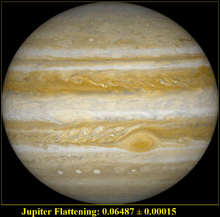The planet Jupiter is an oblate spheroid with flattening ratio 0.06487 ± 0.00015

The oblate spheroid is the approximate shape of many planets and celestial bodies, including Saturn, Jupiter and the quickly-spinning star, Altair; in particular, cartographic and geodetic systems for the Earth are based on a reference ellipsoid. An extreme example of an oblate planet in science fiction is Mesklin, in Hal Clement's novel Mission of Gravity.

### Prolate spheroids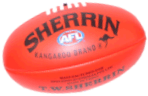An Australian rules football.

The prolate spheroid is the shape of the ball in several sports, such as in rugby football.

Several moons of the Solar system approximate prolate spheroids in shape, though they are actually triaxial ellipsoids. Examples are Mimas, Enceladus, and Tethys (satellites of Saturn) and Miranda (a satellite of Uranus).

In contrast to being distorted into oblate spheroids via rapid rotation, celestial objects distort slightly into prolate spheroids via tidal forces when they orbit a massive body in a close orbit. The most extreme example is Jupiter's moon Io, which becomes slightly more or less prolate in its orbit due to a slight eccentricity, causing spectacular volcanism. It should be noted that the major axis of the prolate spheroid does not run through the satellite's poles in this case, but through the two points on its equator directly facing toward and away from the primary. A famous example in science fiction is Jinx in Larry Niven's Known Space.

It is also used to describe the shape of some nebulae (nebulas) such as the Crab Nebula.

The atomic nuclei of the Actinide elements are shaped like prolate spheroids.

Many submarines have a shape which can be described as prolate spheroid.Wikimedia Commons has media related to Spheroids.Wikisource has the text of the 1911 Encyclopædia Britannica article Spheroid.